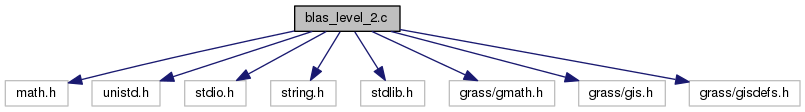GRASS Programmer's Manual  6.5.svn(2014)-r66266
blas_level_2.c File Reference
`#include <math.h>`
`#include <unistd.h>`
`#include <stdio.h>`
`#include <string.h>`
`#include <stdlib.h>`
`#include <grass/gmath.h>`
`#include <grass/gis.h>`
`#include <grass/gisdefs.h>`
Include dependency graph for blas_level_2.c:Go to the source code of this file.

## Macros

#define EPSILON   0.00000000000000001

## Functions

void G_math_Ax_sparse (G_math_spvector **Asp, double *x, double *y, int rows)
Compute the matrix - vector product of sparse matrix **Asp and vector x. More...

void G_math_d_Ax (double **A, double *x, double *y, int rows, int cols)
Compute the matrix - vector product of matrix A and vector x. More...

void G_math_f_Ax (float **A, float *x, float *y, int rows, int cols)
Compute the matrix - vector product of matrix A and vector x. More...

void G_math_d_x_dyad_y (double *x, double *y, double **A, int rows, int cols)
Compute the dyadic product of two vectors. The result is stored in the matrix A. More...

void G_math_f_x_dyad_y (float *x, float *y, float **A, int rows, int cols)
Compute the dyadic product of twMo vectors. The result is stored in the matrix A. More...

void G_math_d_aAx_by (double **A, double *x, double *y, double a, double b, double *z, int rows, int cols)
Compute the scaled matrix - vector product of matrix double **A and vector x and y. More...

void G_math_f_aAx_by (float **A, float *x, float *y, float a, float b, float *z, int rows, int cols)
Compute the scaled matrix - vector product of matrix A and vectors x and y. More...

int G_math_d_A_T (double **A, int rows)
Compute the transposition of matrix A. Matrix A will be overwritten. More...

int G_math_f_A_T (float **A, int rows)

## Macro Definition Documentation

 #define EPSILON   0.00000000000000001

Definition at line 28 of file blas_level_2.c.

## Function Documentation

 void G_math_Ax_sparse ( G_math_spvector ** Asp, double * x, double * y, int rows )

Compute the matrix - vector product of sparse matrix **Asp and vector x.

This function is multi-threaded with OpenMP and can be called within a parallel OpenMP region.

y = A * x

Parameters
 Asp (G_math_spvector **) x (double) *) y (double * )
Returns
(void)

Definition at line 45 of file blas_level_2.c.

Referenced by N_les_integrate_dirichlet_2d(), and N_les_integrate_dirichlet_3d().

 int G_math_d_A_T ( double ** A, int rows )

Compute the transposition of matrix A. Matrix A will be overwritten.

This function is multi-threaded with OpenMP and can be called within a parallel OpenMP region.

Returns 0.

Parameters
 A (double **) rows (int)
Returns
int

Definition at line 371 of file blas_level_2.c.

 void G_math_d_aAx_by ( double ** A, double * x, double * y, double a, double b, double * z, int rows, int cols )

Compute the scaled matrix - vector product of matrix double **A and vector x and y.

z = a * A * x + b * y

This function is multi-threaded with OpenMP and can be called within a parallel OpenMP region.

Parameters
 A (double **) x (double *) y (double *) a (double) b (double) z (double *) rows (int) cols (int)
Returns
(void)

Definition at line 211 of file blas_level_2.c.

 void G_math_d_Ax ( double ** A, double * x, double * y, int rows, int cols )

Compute the matrix - vector product of matrix A and vector x.

This function is multi-threaded with OpenMP and can be called within a parallel OpenMP region.

y = A * x

Parameters
 A (double ** ) x (double *) y (double *) rows (int) cols (int)
Returns
(void)

Definition at line 79 of file blas_level_2.c.

Referenced by N_les_integrate_dirichlet_2d(), and N_les_integrate_dirichlet_3d().

 void G_math_d_x_dyad_y ( double * x, double * y, double ** A, int rows, int cols )

Compute the dyadic product of two vectors. The result is stored in the matrix A.

This function is multi-threaded with OpenMP and can be called within a parallel OpenMP region.

A = x * y^T

Parameters
 x (double *) y (double *) A (float **) – matrix of size rows*cols rows (int) – length of vector x cols (int) – lengt of vector y
Returns
(void)

Definition at line 147 of file blas_level_2.c.

 int G_math_f_A_T ( float ** A, int rows )

Definition at line 404 of file blas_level_2.c.

 void G_math_f_aAx_by ( float ** A, float * x, float * y, float a, float b, float * z, int rows, int cols )

Compute the scaled matrix - vector product of matrix A and vectors x and y.

z = a * A * x + b * y

This function is multi-threaded with OpenMP and can be called within a parallel OpenMP region.

Parameters
 A (float **) x (float *) y (float *) a (float) b (float) z (float *) rows (int) cols (int)
Returns
(void)

Definition at line 293 of file blas_level_2.c.

 void G_math_f_Ax ( float ** A, float * x, float * y, int rows, int cols )

Compute the matrix - vector product of matrix A and vector x.

This function is multi-threaded with OpenMP and can be called within a parallel OpenMP region.

y = A * x

Parameters
 A (float ** ) x (float *) y (float *) rows (int) cols (int)
Returns
(void)

Definition at line 113 of file blas_level_2.c.

 void G_math_f_x_dyad_y ( float * x, float * y, float ** A, int rows, int cols )

Compute the dyadic product of twMo vectors. The result is stored in the matrix A.

This function is multi-threaded with OpenMP and can be called within a parallel OpenMP region.

A = x * y^T

Parameters
 x (float *) y (float *) A (float **= – matrix of size rows*cols rows (int) – length of vector x cols (int) – lengt of vector y
Returns
(void)

Definition at line 177 of file blas_level_2.c.